Author: Oscar Cronquist Article last updated on May 05, 2023

What is the INDEX function?

The INDEX function returns a value from a specific position in a cell range, Excel table, or an array based on a row and column number.

In my opinion this function is one of the most useful functions in Excel .

There are two ways to use the INDEX function: array form or reference form.

What is the difference between the INDEX function and the OFFSET function?

The OFFSET function is also able to extract a 2D range from a given cell range which the INDEX function can't as far as I know.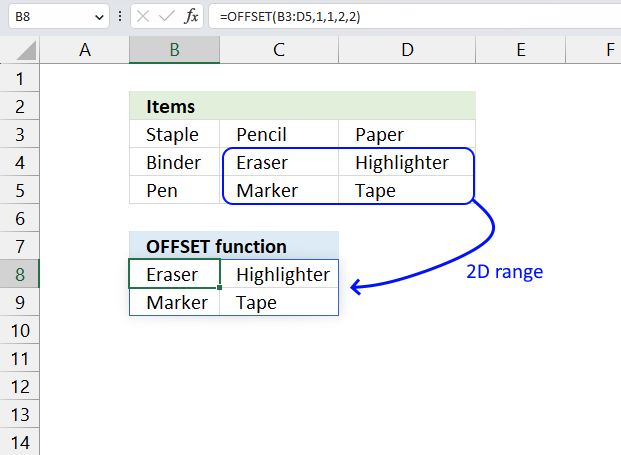However, the OFFSET function is volatile meaning it recalculates more often than non-volatile functions. Your Excel worksheet could become significantly slower if you use the OFFSET function frequently.

I recommend using the INDEX function over the OFFSET function as much as possible because of this disadvantage.

What if you compare the new Excel 365 FILTER function with the INDEX function?

The new FILTER function lets you create much smaller formulas and is really powerful. It replaces the INDEX function in lots of situations. Check out the FILTER function examples.

Can the INDEX function return multiple values?

Yes, it can. Excel versions earlier than Excel 365 need to enter the formula as an array formula for it to work. This is what the INDEX function can do:

• Return all values in a given row or column. See section 6, section 9.1 and 9.2
• Return all values in a given cell range. See section 8.
• A formula that return a single value, however, the formula is constructed so it returns a new value in each cell. This technique is now more or less obsolete because of the new FILTER function and other new Excel 365 functions.

What is the difference between the INDEX function and the VLOOKUP function?

The INDEX function and the VLOOKUP function are both used to look up values in a table or a range of cells, but they have some differences.

The VLOOKUP function looks up a value in the first column of a range and returns a value from another column in the same row. The column number is specified by a number argument.
For example, =VLOOKUP(A2,B2:D10,3,FALSE) will look up the value in A2 in the first column of B2:D10 and return the value from the third column (column D) in the same row.

The INDEX function returns a value from a specific row and column in a range. The row and column numbers are specified by separate arguments.
For example, =INDEX(B2:D10,4,2) will return the value from the fourth row and second column (cell C5) in the range B2:D10.

One advantage of the INDEX function over the VLOOKUP function is that it can look up values in any column of a range, not just the first one. Another advantage of the VLOOKUP function over the INDEX function is that it is simpler to use and learn and requires fewer arguments, however, the INDEX and MATCH functions are more versatile.

## 1. INDEX Function Syntax

### Array form

INDEX(array, [row_num], [column_num])

### Reference form

INDEX(array, [row_num], [column_num], [area_num])

## 2. INDEX Function Arguments

### Array form

 array Required. The cell range you want to get a value from. You can also use an array. [row_num] Optional. The relative row number of a specific value you want to get. If omitted the INDEX function returns all values if you enter it as an array formula. Update! The 365  subscription version of Excel returns all values without needing to enter the formulas an array formula. [column_num] Optional. The relative column number of a specific value you want to get. If omitted the INDEX function returns all values if you enter it as an array formula. Update! The 365  subscription version of Excel returns all values without needing to enter the formulas an array formula. [area_num] Optional. A number representing the relative position of one of the ranges in the first argument.

### Reference form

 cell reference Required. A reference a cell range or multiple cell ranges. [row_num] Optional. The relative row number of a specific value you want to get. If omitted the INDEX function returns all values if you enter it as an array formula. Update! The 365  subscription version of Excel returns all values without needing to enter the formulas an array formula. [column_num] Optional. The relative column number of a specific value you want to get. If omitted the INDEX function returns all values if you enter it as an array formula. Update! The 365  subscription version of Excel returns all values without needing to enter the formulas an array formula. [area_num] Optional. A number representing the relative position of one of the ranges in the first argument.

What is the difference between the array form and the reference form?

The difference between the array form and the reference form of the INDEX function is that the reference form allows more than one array, you also need to use optional argument [area_num] to specify which array should be used.

The array form only allows one array or range as the first argument, however, this is how the INDEX function is most used.. Both forms return a value or a reference based on a given row and column location.

Use the reference form when you have multiple ranges and you want to switch between them based on a condition. Read this section on how to use the reference form:
How to use the [area_num] argument - INDEX function

## 3. INDEX function Example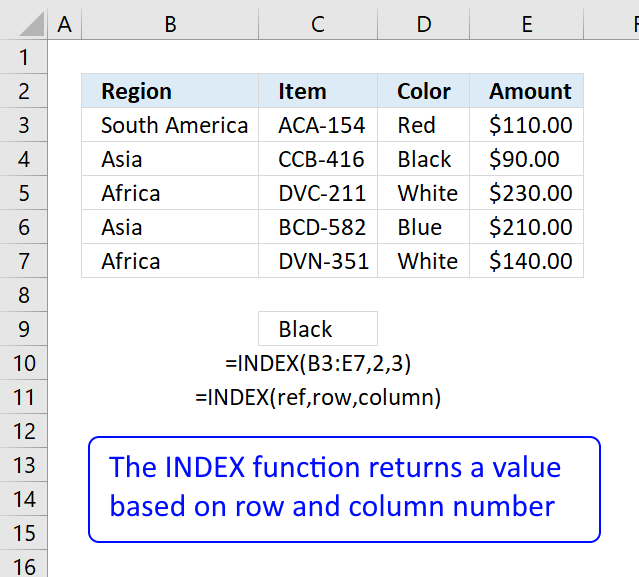Formula in cell C9:

=INDEX(B3:E7,2,3)

This formula returns a value from row 2 and column 3 based on cell range B3:E7, note these are relative positions.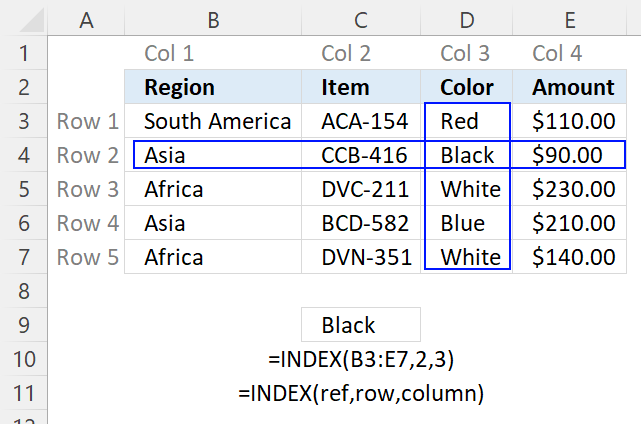The image above shows the relative row and column numbers, row 2 and column 3 are highlighted. The intersection of those two is the value the INDEX function returns.

## 4. How to create array constants in the INDEX function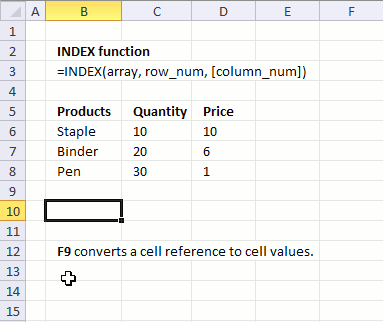The first argument in the INDEX function is array or a cell reference to a cell range. What is an array constant? An array constant is a set of values hardcoded into the formula.

To demonstrate in greater detail what an array of constants is you can convert a cell reference to an array of constants:

1. Select the cell reference.
2. Press F9 to convert the cell reference to values, see the animated image above.
When you convert a cell range to constants Excel automatically creates double quotes around text values, however, note that numbers are not changed.

B6:D8

becomes

{"Staple",10,10;"Binder",20,6;"Pen",30,1}

and each value is separated by a delimiting character. Comma (,) is used to separate columns and semicolon (;) to separate rows.

The English language version of excel uses commas and semicolons, other language versions of excel may use other characters. You can change this in the Regional settings in Windows.

Here is an example of  array constants used in an INDEX function:

=INDEX({"Staple", 10, 10; "Binder", 20, 6; "Pen", 30, 1}, 3, 1)

The greatest disadvantage of using an array is that you need to edit the formula if you need to change one of the values in the array, contrary to a cell reference.

Here is an example of a cell reference being used in an INDEX function:

=INDEX(B6:D8, 3, 1)

You don't need to edit this formula if one of the values in cell range B6:D8 is changed, the formula is using the new value automatically.

Remember that relative cell references (B6:D8) changes when you copy the cell and paste to cells below. Absolute cell references (\$B\$6:\$D\$8) do not change when the cell is copied to cells below.

Replace a formula with its result

## 5. How to get a value in a given cell range based on a row number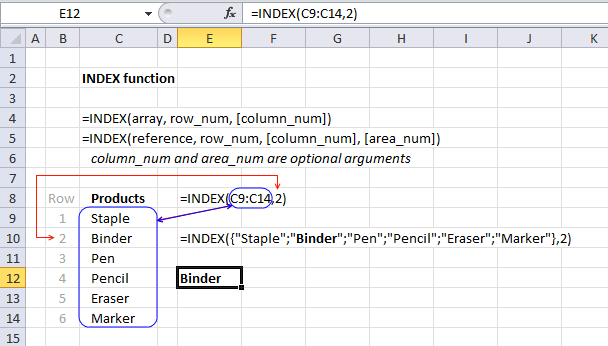The second argument in the INDEX function is the row_num which you are required to enter, the [column_num] argument is optional however hence the brackets. It allows you to choose the row in an array or cell range, from which to return a value.

If you use an array or cell range with values distributed in one column only there is no need to use the second optional argument which specifies the column, there is only one column to use. Here is an example of an array containing values in a single column, no comma as a delimiting value in this array which would have indicated that there would have been multiple columns.

=INDEX({"Staple"; "Binder"; "Pen"; "Pencil"; "Eraser"; "Marker"}, 2)

The following formula uses a cell reference instead of hardcoded values:

=INDEX(C9:C14, 2)

Cell range C9:C14 has values separated by a semicolon. The cell range is one-dimensional. In this example, the value from the second row will be returned, see image above.

## 6. How to return all values in a row or column in a given cell range with the INDEX function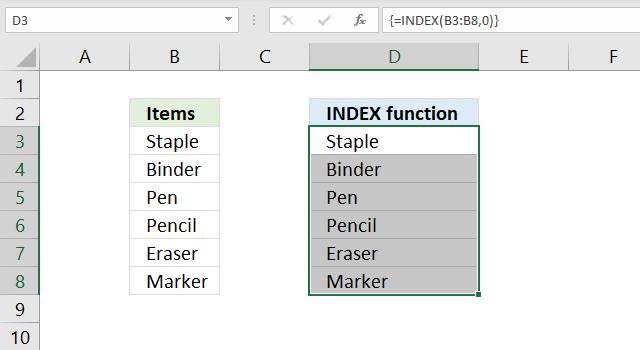It is also possible to return an array of values if you omit or use a zero as row_num argument:

=INDEX(C9:C14,)
=INDEX(C9:C14,0)

Both these formulas return an array of values. To be able to display all values you need to enter the formula as an array formula in a cell range that has the same number of cells as the cell range or values in the array.

1. Select cell range D3:D8.
2. Type the formula =INDEX(C9:C14,0)
3. Press and hold CTRL + SHIFT simultaneously.
4. Press Enter once.
5. Release all keys.

The formula in the formula bar changes to {=INDEX(C9:C14,0)}, do not add these curly brackets yourself, they appear automatically. See the image above.

Update 1/22/2020!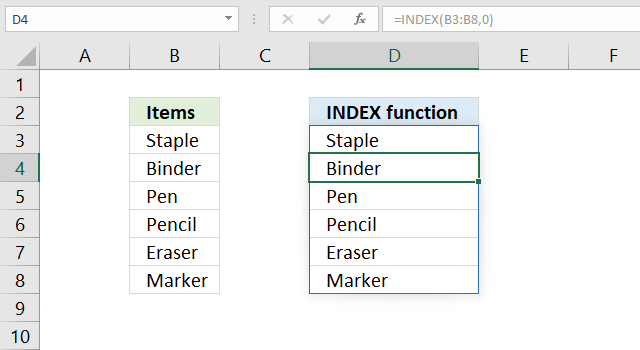Excel users owning Excel 365 subscription version now have the option to not enter the formula as an array formula but as a regular formula. They are called dynamic arrays and behaves differently than array formulas. Array formulas can still be used in order to be compatible with earlier Excel versions, however, Microsoft suggests that you should from now on use dynamic arrays instead of array formulas.

The formula is entered as a regular formula and extends automatically if the cells needed below are empty, this is called spilling by Microsoft. The remaining cells show a greyed out formula in the formula bar, only the first cell contains a formula in black.

The blue border around the cell range indicates that the cell range contains a spilled formula and disappears when you press with left mouse button on a cell outside the range.

## 7. Get a value in a given cell range based on a row and column number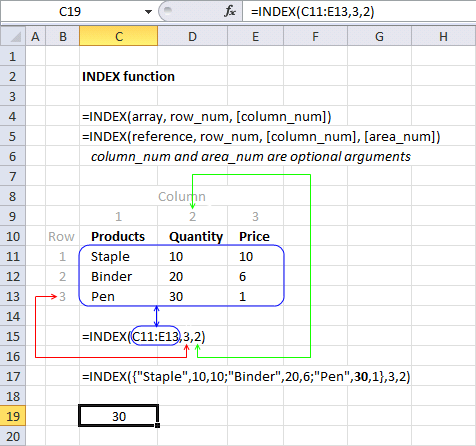The column_num argument allows you to choose a column from which to return a value. This argument is optional, for example, if you only have values in a single column.

The cell range C11:E13 is two-dimensional meaning there are multiple rows and columns. In this example, the value in the third row and the second column is returned.

=INDEX(C11:E13, 3, 2)

I have greyed out the row and column numbers in the image above, this makes it easier to see that value 30 is where row 3 and column 2 interesects.

The following formula contains an array of constants. It retrieves the value from the third row and second column.

=INDEX({"Staple", 10, 10; "Binder", 20, 6; "Pen", 30, 1}, 3, 2)

{"Staple", 10, 10; "Binder", 20, 6; "Pen", 30, 1} has values separated by commas and semicolons meaning commas separate values between columns and semicolons separate values between rows.

## 8. How to use the [area_num] argument - INDEX function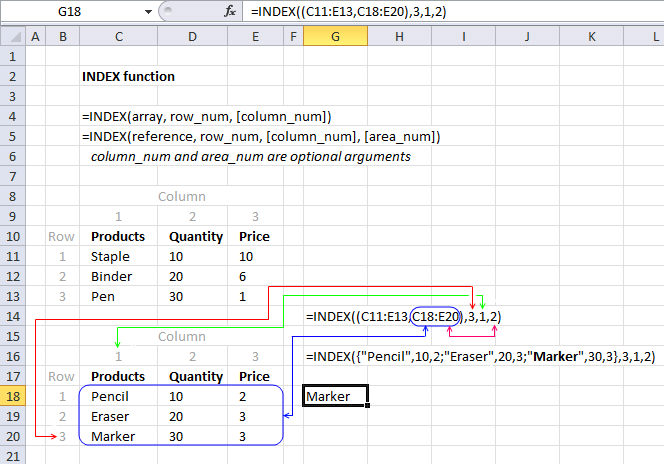The INDEX function lets you have multiple cell references in the first argument, the area_num argument allows you to pick a cell range in the reference argument.

INDEX(referencerow_num[column_num], [area_num])

The following formula has two references pointing to two different cell ranges.

=INDEX((C11:E13,C18:E20),3,1,2)

The area_num selects from which cell reference to return a value. In this example, area_num is two therefore the second cell reference is used. The item in the third row and the first column is returned.

The INDEX function returns a #VALUE! error if you reference a cell range on another worksheet, however, there is a workaround.

## 9. How to return the entire row in a given cell range using the INDEX function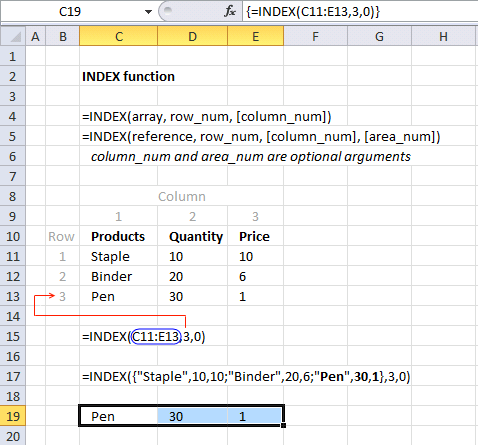The INDEX function is also capable of returning an array from a column, row, and both columns and rows. The following formula demonstrates how to extract all values from row three:

=INDEX(C11:E13,3,0)

The formula in cell C19:E19 is an array formula, here is how to enter an array formula.

Excel 365 users can ignore these steps, enter the formula as a regular formula. It will spill values to cells below and to the right automatically.
1. Select cell range C19:E19.
2. Type =INDEX(C11:E13,3,0) in formula bar.
3. Press and hold CTRL + SHIFT simultaeously.
4. Press Enter.
5. Release all keys.

### 9.1 How to return an column of values in a given cell range using the INDEX function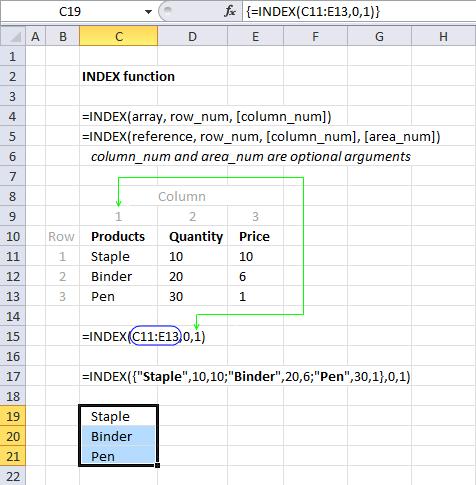The example above demonstrates an array formula that returns all values from column 1 from cell range C11:E13.

=INDEX(C11:E13, 0, 1)

### 9.2 How to return the entire specified cell range  - INDEX function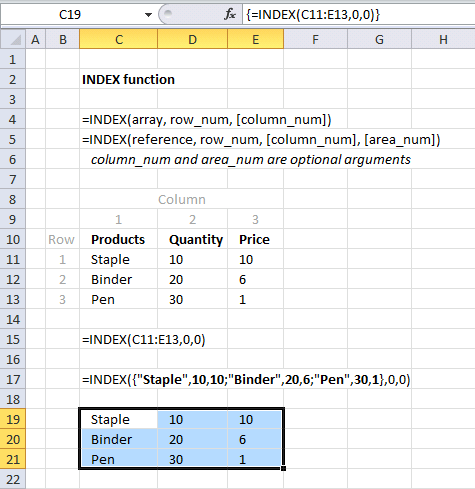The example above returns all values from a two-dimensional cell range. The following array formula returns all values on all rows and columns from a cell range.

=INDEX(C11:E13,0,0)

The zeros in both the row_num and column_num arguments allow you to get all values in the specified cell range. The example above uses C11:E13 as the array argument, all values in C11:E13 are returned.

## 10. How to build a dynamic cell reference using the INDEX function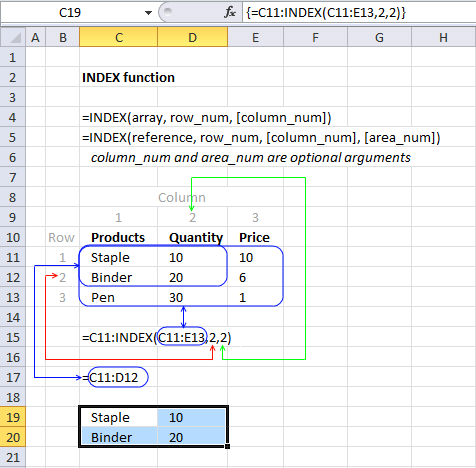The INDEX function can also be used to create a cell reference, for example, a dynamic range created by a formula in a named range.

Array formula in cell range C19:D20:

=C11:INDEX(C11:E13,2,2)

### Explaining formula

#### Step 1 - Calculate cell reference

INDEX(C11:E13,2,2)

returns cell reference D12.

#### Step 2 - Build new cell reference

The colon lets you append two single cell references creating a larger cell range reference.

C11:INDEX(C11:E13,2,2)

becomes

C11:D12

and returns

{"Staple",10;"Binder",20}.

### Final note

There are some magic things you can do with the array argument if you use an Excel version prior to Excel 365. See this post: No more array formulas?

## 11. INDEX function errors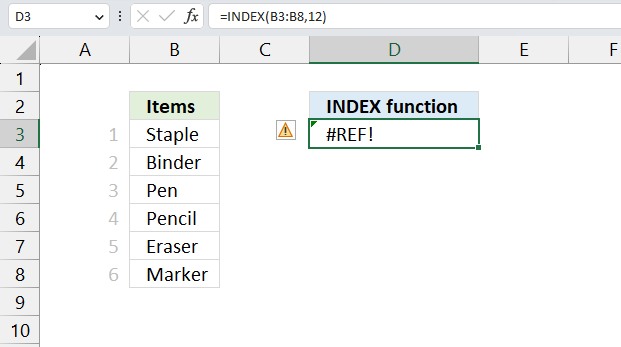The INDEX returns a #REF! error if the row_num or col_num arguments points to a cell outside the given cell reference. Change the row_num or col_num so it points to a cell insdie the given array argument.

The example above shows the INDEX function in cell D3, it returns #REF error because the row_num points to a cell outside cell range B3:B8. Cell range B3:B8 contains six cells, the row_num argument points to cell 12.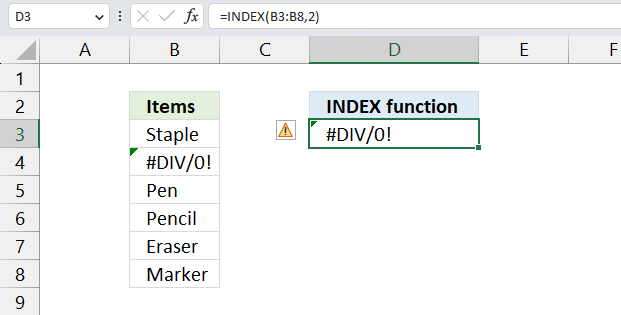An error is shown if the source cell contains an error value. The image above shows the INDEX function in cell D3, it retrieves the value from cell B4, however, B4 contains a #DIV/0 error. The INDEX function in cell D3 returns the same error.

Make sure your source data is free from errors.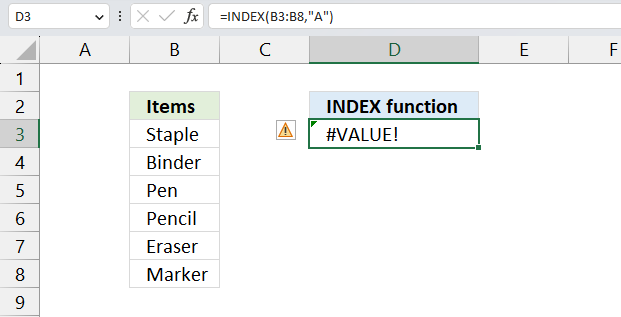The INDEX function returns a #VALUE! error if the number of arguments are wrong or the arguments contain text values. The image above shows the INNDEX function in cell D3 returning a #VALUE! error. The second argument row_num is a text value and not a number which is not valid.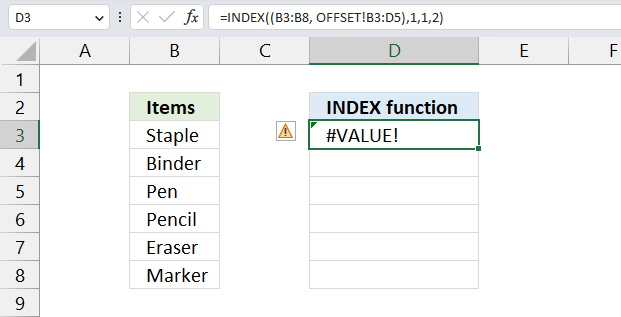The INDEX function returns a #VALUE! error if a reference points to a cell range located on another worksheet. The image above shows the INDEX function in cell D3, the first cell reference points to B3:B8, however, the second cell reference points to cell range B3:D5 on another worksheet. This is not allowed, a workaround is required.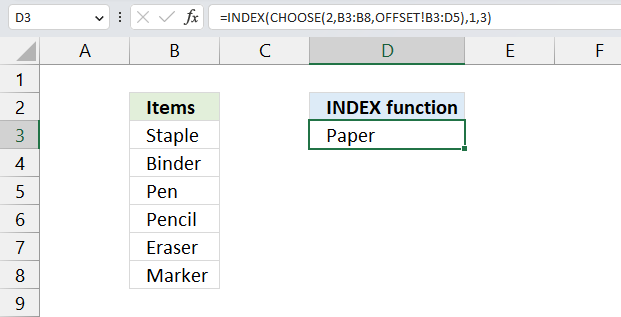The image above shows the workaround in cell D3, this formula allows you to use cell references pointing to other worksheets.

Formula in cell D3:

=INDEX(CHOOSE(2,B3:B8,OFFSET!B3:D5),1,3)

### 11.1 Explaining formula

#### Step 1 - Select cell reference

The CHOOSE function gets a value based on a number.

Function syntax: CHOOSE(index_num, value1, [value2], ...)

CHOOSE(2,B3:B8,OFFSET!B3:D5)

returns

OFFSET!B3:D5. This is a cell reference on a worksheet named OFFSET.

#### Step 2 - Get value based on selected cell reference

INDEX(CHOOSE(2,B3:B8,OFFSET!B3:D5),1,3)

becomes

INDEX(OFFSET!B3:D5,1,3)

and returns "Paper".

The INDEX function gets the value on row 1 and column 3 in cell range OFFSET!B3:D5.

## 12. Can you replace the VLOOKUP function with the INDEX function?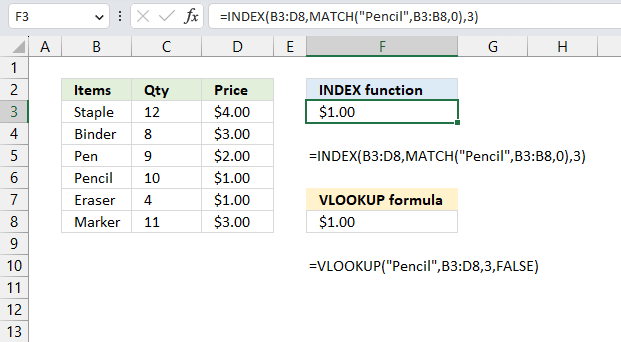Yes, you can. The image above shows the INDEX formula in cell F3 and the VLOOKUP formula in cell F8. The INDEX formula needs the MATCH function to determine the relative position of value "Pencil" in cell range B3:B8.

The VLOOKUP finds the position automatically and the formula is somewhat smaller and easier to understand, the downside is that the VLOOKUP function performs a lookup only in the leftmost column. However, the INDEX formula can easily be customized to perform a lookup in any column you like.

Formula in cell F8:

=VLOOKUP("Pencil",B3:D8,3,FALSE)

Formula in cell F3:

=INDEX(B3:D8,MATCH("Pencil",B3:B8,0),3)

This example demonstrates that the INDEX formula above replaces the VLOOKUP formula, it performs the exact same task. This lets you compare the formulas and see the differences.

## 13. Where do I use the INDEX - MATCH functions instead of VLOOKUP?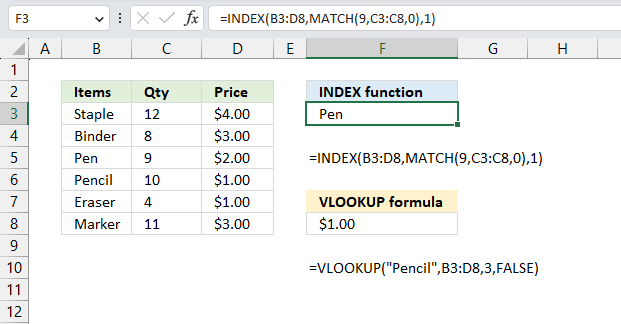Use the INDEX - MATCH functions when you want to lookup a value not in the leftmost column of a cell range, the VLOOKUP function can only perform a lookup in the leftmost column of the specified cell range. The image above shows an example in cell F3.

=INDEX(B3:D8,MATCH(9,C3:C8,0),1)

The formula looks for number 9 in column "Qty" and finds it on row 3 in cell range C3:C8, this range is not the leftmost column in cell range B3:D8. The INDEX function retrieves the value from row 3 and column in B3:C8 which is "Pen".

### Explaining formula

#### Step 1 - Calculate the relative position of number 9 in C3;C8

The MATCH function returns the relative position of an item in an array that matches a specified value in a specific order.

Function syntax: MATCH(lookup_value, lookup_array, [match_type])

MATCH(9,C3:C8,0)

becomes

MATCH(9,{12;8;9;10;4;11},0)

and returns 3. 9 has the relative position three in the array.

#### Step 2 - Get value

The INDEX function returns a value or reference from a cell range or array, you specify which value based on a row and column number.

Function syntax: INDEX(array, [row_num], [column_num])

INDEX(B3:D8,MATCH(9,C3:C8,0),1)

becomes

INDEX({"Staple",12,4;
"Binder",8,3;
"Pen",9,2;
"Pencil",10,1;
"Eraser",4,1;
"Marker",11,3},3,1)

and returns "Pen" in cell F3.